# Periodic function

(diff) ← Older revision | Latest revision (diff) | Newer revision → (diff)

A function having a period (cf. Period of a function).

Let a functionbe defined on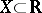and have period. To obtain the graph ofit is sufficient to have the graph ofon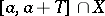, whereis a certain number, and shift it alongover. If a periodic functionwith periodhas a finite derivative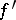, thenis a periodic function with the same period. Letbe integrable over any segment and have period. The indefinite integral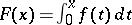has periodif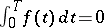, otherwise it is non-periodic, such as for example for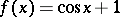, where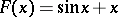.

A.A. Konyushkov

A periodic function of a complex variableis a single-valued analytic functionhaving only isolated singular points (cf. Singular point) in the complex-planeand for which there exists a complex number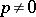, called a period of the function, such that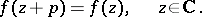Any linear combination of the periods of a given periodic functionwith integer coefficients is also a period of. The set of all periods of a given periodic function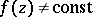constitutes a discrete Abelian group under addition, called the period group of. If the basis of this group consists of one unique basic, or primitive, period, i.e. if any periodis an integer multiple of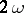, thenis called a simply-periodic function. In the case of a basis consisting of two basic periods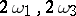,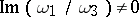, one has a double-periodic function. If the periodic functionis not a constant, then a basis of its period group cannot consist of more than two basic independent periods (Jacobi's theorem).

Any strip of the form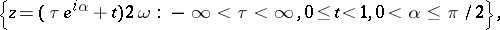whereis one of the basic periods ofor is congruent to it, is called a period strip of; one usually takes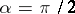, i.e. one considers a period strip with sides perpendicular to the basic period. In each period strip, a periodic function takes any of its values and moreover equally often.

Any entire periodic functioncan be expanded into a Fourier series throughout: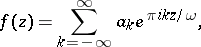(*)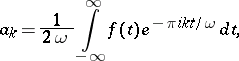which converges uniformly and absolutely on the straight line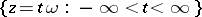and, in general, on any arbitrarily wide strip of finite width parallel to that line. The case when an entire periodic functiontends to a certain finite or infinite limit at each of the two ends of the period strip is characterized by the fact that the series (*) contains only a finite number of terms, i.e.should be a trigonometric polynomial.

Any meromorphic periodic functionthroughoutwith basic periodcan be represented as the quotient of two entire periodic functions with the same period, i.e. as the quotient of two series of the form (*). In particular, the class of all trigonometric functions can be described as the class of meromorphic periodic functions with periodthat in each period strip have only a finite number of poles and tend to a definite limit at each end of the period strip.

How to Cite This Entry:
Periodic function. Encyclopedia of Mathematics. URL: http://encyclopediaofmath.org/index.php?title=Periodic_function&oldid=15049
This article was adapted from an original article by A.A. Konyushkov, E.D. Solomentsev (originator), which appeared in Encyclopedia of Mathematics - ISBN 1402006098. See original article